TitleCollege Algebra
Answer/Discussion to Practice Problems
Tutorial 10: Adding and Subtracting Rational ExpressionsAnswer/Discussion to 1a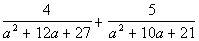Step 1: Find the LCD as shown in tutorial 10.

 The first denominator has the following two factors:*Factor the trinomial

 The second denominator has the following two factors: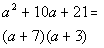*Factor the trinomial

 Putting all the different factors together and using the highest exponent, we get the following LCD: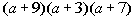Step 2: Write equivalent fractions using the LCD if needed.

 Rewriting the first expression with the LCD: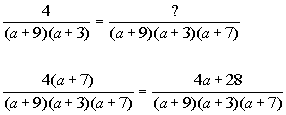*Missing the factor of (a + 7) in the den. *Mult. top and bottom by (a + 7)

 Rewriting the second expression with the LCD: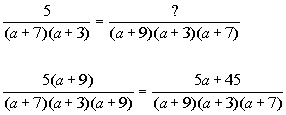*Missing the factor of (a + 9) in the den. *Mult. top and bottom by (a + 9)

 Step 3: Combine the rational expressions as shown in tutorial 10.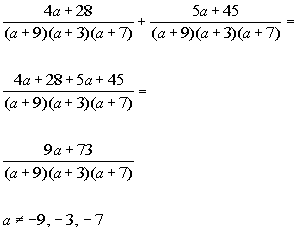*Combine the numerators *Write over common denominator

 Step 4: Reduce to lowest terms.

 This rational expression cannot be simplified down any farther.

 Also note that the values that would be excluded from the domain are -9, -3 and -7.  These are the values that make the original denominator equal to 0.Answer/Discussion to 1b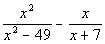Step 1: Find the LCD as shown in tutorial 10 if needed.

 The first denominator has the following two factors: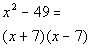*Factor the difference of squares

 The second denominator has the following factor:Putting all the different factors together and using the highest exponent, we get the following LCD: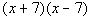Step 2: Write equivalent fractions using the LCD if needed.

 Since the first rational expression already has the LCD, we do not need to change this fraction.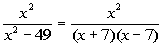*Rewriting denominator in factored form

 Rewriting the second expression with the LCD: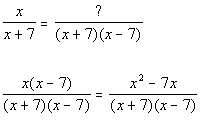*Missing the factor of (x - 7) in the den. *Mult. top and bottom by (x - 7)

 Step 3: Combine the rational expressions as shown in tutorial 10.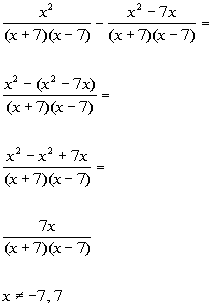*Combine the numerators *Write over common denominator   *Distribute the minus sign through the (   )

 Step 4: Reduce to lowest terms.

 This rational expression cannot be simplified down any farther.

 Also note that the values that would be excluded from the domain are -7 and 7.  These are the values that make the original denominator equal to 0.

Last revised on Dec. 15, 2009 by Kim Seward.
All contents copyright (C) 2002 - 2010, WTAMU and Kim Seward. All rights reserved.